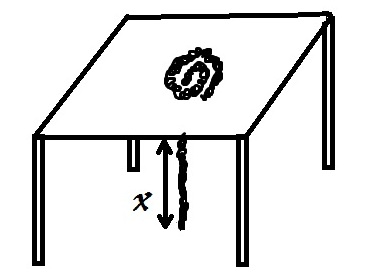# The Inelastic ChainA chain of mass per unit length $\displaystyle\lambda$ is piled on top of a table. A small hole is cut, through which the chain starts falling. Assume that the only moving part of the rope is that which hangs through the hole, beneath the table. The section of rope on the table is at rest.

Find the velocity of the chain at $\displaystyle t = 2$ seconds.

Details and Assumptions

• $\displaystyle \lambda = 0.5kg/m$
• $\displaystyle g = 9.8m/s^2$
×

Problem Loading...

Note Loading...

Set Loading...